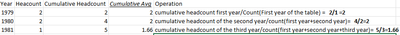cancel
Showing results for
Did you mean:New Member

Hi Everyone!

Sorry if my english is not good.

I want to calculate cumulative average headcount.

I have two tables

The Data_Table have the records of employees(termination date(fecha de baja [fix]), Hiredate(fecha de ingreso [fix]))

The Calendario_Table have the date (1/1/1979-12/31/2026)

I have related two fields with date table(terminationdate,hiredate)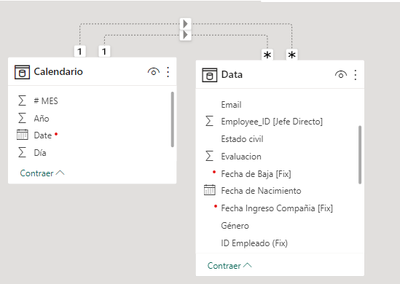First, I created a measure to calculate the headcountAfter, I created a measure to calculate the cumulative headcount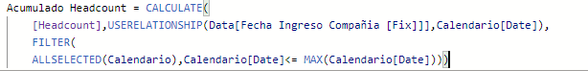But when I created the measure for the cumulative average, the result is not correct.

But i dont know  where i am wrong.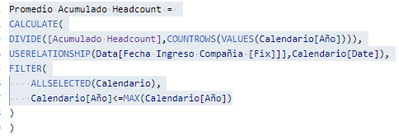The correct cumulative avg is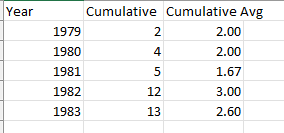Could you tell me how I can solve it?

Thank you.

5 REPLIES 5Resolver I

Try replacing "Date" inside allselected with "Date[year]" because for me with similar kind of data these below 2 measures are working fine.
1)

Cummulative count =
CALCULATE(
[Order Count],
FILTER(
ALLSELECTED('Date'),
'Date'[Year] <= MAX('Date'[Year])
)
)

2)
cumulative average =
Var a =     FILTER(
ALLSELECTED('Date'[Year]), ---- in your case calendario with calendario[Ano]
'Date'[Year] <= MAX('Date'[Year])
)

return DIVIDE([Cummulative count], COUNTROWS(a))New Member

I tried the measure.The measure does work when I take the date from the same table where the data is.

But when I take the date from the calendar table, the measure doesnt work.

It should work, because is the same step,only I add the instruction  userelationship to connect the tables, but it doesn´t work

Could I share the exercise with you by email?

We could review the exercise together.

Thanks!Resolver I

Email -- aniketraut0077@gmail.comResolver INew Member

Hi

Thanks

I changed the numerator but the result is the sameI am trying to do this operation